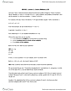# MATA02H3 Lecture Notes - Asymptote, Richter Magnitude Scale, Logarithm

108 views2 pages
School
Department
Course
Professorraspberryleopard199 and 38930 others unlocked12
Verified Note
12 documents

## Document Summary

The y-values all grow quickly to the right of (0,1) The y-values get smaller and nearer to 0 to the left of (0,1). The larger the base, the quicker the y-value to the left approach the asymptote. The base of 1 is a horizontal line. (1/2)^x (1/3)^x (1/4)^x (1/5)^x. The y-values all grow quickly to the left of (0,1) The y-values get smaller and nearer to 0 to the right of (0,1). The formula for exponential doubling is n(t) = n02^t/d. N0- the initial number or concentration at time 0. 2- because the population is doubling t- time elapsed since measuring started (express this in the same units as d) d- doubling period. C0- the initial number or concentration at time 0. 2^-1 or 1/2- because the population is halving t- time elapsed since measuring started (express this in the same units as h) d- half life. A- the amount the account will grow to.